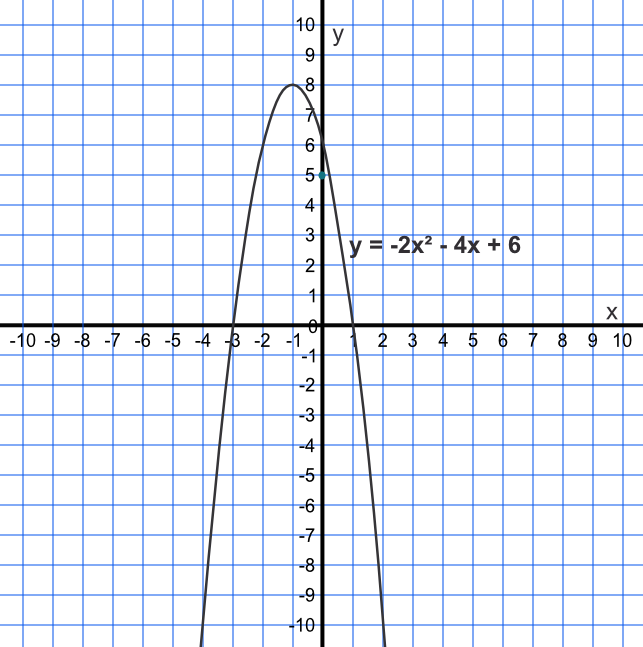If the coefficient (the multiple) of the x^2 term is positive, then the turning point is a minimum. If negative, the turning point is a maximum.

A quadratic curve is vertically symmetrical about its turning point, or vertex.

The x-value for the turning point is given by -frac(b)(2a) for a function ax^2+bx+c. Substituting this value into the equation gives the y-value.

## Example 1

What are the coordinates for the turning point for the equation y = -2x^2 - 4x + 6?

The x-value is given by -frac(b)(2a) = -frac(-4)(2 xx -2) = -1.

Substituting -2(-1)^2 - 4(-1) + 6 = -2 + 4 + 6 = 8